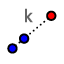# Exploring Dilations with different centers

Topic:
Dilation

## DIRECTIONS:

1) Select the slider tool to create a slider. Name the slider k. Set Min = -5, Max = 5, Increment = 0.1 2) Select the DILATE FROM POINTtool. Highlight a box around point A, point B, and Curious George's picture. Then select point C (to serve as center of dilation) In the pop-up box that appears, enter "k" (without the " " 's) to serve as the scale factor. 3) Select the Move tool. Explore! Please answer the questions that appear below the applet.

## 4)

Suppose point P = (a, b) is dilated about (0,0) with scale factor k. What would the coordinates of the image of P be? Express these coordinates in terms of a, b, and/or k.

## 5)

Suppose point P = (a, b) is dilated about (c, d) with scale factor k. What would the coordinates of the image of P be? Express these coordinates in terms of c, d, a, b and/or k.

When you're done (or if you're unsure of something), feel free to check by watching the quick silent screencast below the applet.

## Quick (Silent) Demo

What do you think you were expected to learn from this exploration?